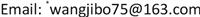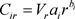﻿ 基于学习与恶化效应的松弛工期指派排序问题 Slack Due Date Assignment Scheduling Problem Based on Learning and Deterioration Effect

Operations Research and Fuzziology
Vol. 10  No. 01 ( 2020 ), Article ID: 33685 , 6 pages
10.12677/ORF.2020.101003

Slack Due Date Assignment Scheduling Problem Based on Learning and Deterioration Effect

Xun Xi, Xiaona Sun, Chong Wang, Hui Xu, Honglin Pan, Jibo Wang*

School of Science, Shenyang Aerospace University, Shenyang LiaoningReceived: Dec. 6th, 2019; accepted: Dec. 20th, 2019; published: Dec. 30th, 2019ABSTRACT

This paper considers a scheduling problem with learning and deterioration effect; under a single machine and slack due date assignment, our objective is to determine the schedule of jobs and the common slack flow in order to minimize the linear combination of the just-in-time cost (including the earliness, tardiness and common slack flow) and makespan . The properties of the optimal solution are given, and then we prove that the problem can be solved in polynomial time.

Keywords:Scheduling, Deterioration Effect, Learning Effect, Due Date Assignment, Single-Machine1. 引言

2. 问题描述

n个工件 $J=\left\{{J}_{1},{J}_{2},\cdots ,{J}_{n}\right\}$ 要在一台机器上加工，同一时刻这台机器最多加工一个工件，且不允许中断。假设工件 ${J}_{i}$ 的正常加工时间为 ${a}_{i}$，则工件 ${J}_{i}$ 的实际加工时间为

${p}_{i}^{A}={a}_{i}{r}^{{b}_{i}}+\alpha t$,$i=1,2,\cdots ,n$,

$Z\left(\pi ,{q}_{opt}\right)=\eta \left(\underset{i=1}{\overset{n}{\sum }}{\omega }_{i}|{C}_{\pi \left(i\right)}-{d}_{\pi \left(i\right)}|+{\omega }_{0}{q}_{opt}\right)+\left(1-\eta \right){C}_{\mathrm{max}}$

$1|SLK,{q}_{opt},{p}_{i}^{A}={a}_{i}{r}^{{b}_{i}}+\alpha t|\eta \left(\underset{i=1}{\overset{n}{\sum }}{\omega }_{i}|{C}_{\pi \left(i\right)}-{d}_{\pi \left(i\right)}|+{\omega }_{0}{q}_{opt}\right)+\left(1-\eta \right){C}_{\mathrm{max}}$

Liu等  证明了工件加工时间为常数下的问题 $1|SLK,{q}_{opt}|\underset{i=1}{\overset{n}{\sum }}{\omega }_{i}|{C}_{\pi \left(i\right)}-{d}_{\pi \left(i\right)}|+{\omega }_{0}{q}_{opt}$ 是多项式时间可解的，下面我们证明问题 $1|SLK,{q}_{opt},{p}_{i}^{A}={a}_{i}{r}^{{b}_{i}}+\alpha t|\eta \left(\underset{i=1}{\overset{n}{\sum }}{\omega }_{i}|{C}_{\pi \left(i\right)}-{d}_{\pi \left(i\right)}|+{\omega }_{0}{q}_{opt}\right)+\left(1-\eta \right){C}_{\mathrm{max}}$ 仍然多项式时

3. 主要结论

${q}_{opt}={C}_{\pi \left(l\right)}={\sum }_{i=0}^{l}{p}_{\pi \left(i\right)}^{A}$，其中l是序列 ${\omega }_{0},{\omega }_{1},\cdots ,{\omega }_{n}$ 的中位数，并使下面不等式成立

$\underset{j=0}{\overset{l}{\sum }}{\omega }_{j}\le \underset{j=l+1}{\overset{n}{\sum }}{\omega }_{j},\underset{j=0}{\overset{l+1}{\sum }}{\omega }_{j}\ge \underset{j=l+2}{\overset{n}{\sum }}{\omega }_{j}$ (1)

${\lambda }_{i}=\left\{\begin{array}{l}\eta \underset{v=0}{\overset{i}{\sum }}{w}_{v}-\eta +1,i=1,2,\cdots ,l;\\ \eta \underset{v=i+1}{\overset{n}{\sum }}{\omega }_{v}-\eta +1,i=l+1,\cdots ,n-1;\\ 1-\eta ,i=n\end{array}$ (2)

$\begin{array}{l}\eta \left(\underset{i=1}{\overset{n}{\sum }}{w}_{i}|{C}_{\pi \left(i\right)}-{d}_{\pi \left(i\right)}|+{w}_{0}{q}_{opt}\right)+\left(1-\eta \right){C}_{\mathrm{max}}\\ =\eta \left({\omega }_{0}{C}_{\pi \left(l\right)}+\underset{i=1}{\overset{l+1}{\sum }}{\omega }_{i}\left({C}_{\pi \left(l\right)}-{C}_{\pi \left(i-1\right)}\right)+\underset{i=l+2}{\overset{n}{\sum }}{\omega }_{i}\left({C}_{\pi \left(i-1\right)}-{C}_{\pi \left(l\right)}\right)\right)+\left(1-\eta \right){C}_{\mathrm{max}}\\ =\eta \left(\underset{i=0}{\overset{l+1}{\sum }}{\omega }_{i}\underset{v=i}{\overset{l}{\sum }}{p}_{\pi \left(v\right)}^{A}+\underset{i=l+2}{\overset{n}{\sum }}{\omega }_{i}\underset{v=l+1}{\overset{i-1}{\sum }}{p}_{\pi \left(v\right)}^{A}\right)+\left(1-\eta \right)\underset{i=1}{\overset{n}{\sum }}{p}_{\pi \left(i\right)}^{A}\\ =\underset{i=1}{\overset{n}{\sum }}{\lambda }_{i}{p}_{\pi \left(i\right)}^{A}\end{array}$

$\begin{array}{l}{p}_{\pi \left(i\right)}^{A}=\alpha {\left(1+\alpha \right)}^{i-2}{a}_{{\pi }_{\left(1\right)}}{1}^{{b}_{{\pi }_{\left(1\right)}}}+\alpha {\left(1+\alpha \right)}^{i-3}{a}_{{\pi }_{\left(2\right)}}{2}^{{b}_{{\pi }_{\left(2\right)}}}+\cdots +\alpha \left(1+\alpha \right){a}_{{\pi }_{\left(i-2\right)}}{\left(i-2\right)}^{{b}_{{\pi }_{\left(i-2\right)}}}\\ \text{}+\alpha {a}_{{\pi }_{\left(i-1\right)}}{\left(i-1\right)}^{{b}_{{\pi }_{\left(i-1\right)}}}+{a}_{{\pi }_{\left(i\right)}}{i}^{{b}_{{\pi }_{\left(i\right)}}}\end{array}$

$\begin{array}{c}Z\left(\pi ,{q}_{opt}\right)=\eta \left(\underset{i=1}{\overset{n}{\sum }}{w}_{i}|{C}_{\pi \left(i\right)}-{d}_{\pi \left(i\right)}|+{w}_{0}{q}_{opt}\right)+\left(1-\eta \right){C}_{\mathrm{max}}\\ =\underset{i=1}{\overset{n}{\sum }}{\lambda }_{i}{p}_{\pi \left(i\right)}^{A}\\ ={a}_{{\pi }_{\left(1\right)}}{1}^{{b}_{{\pi }_{\left(1\right)}}}\left({\lambda }_{1}+\alpha {\lambda }_{2}+\alpha \left(1+\alpha \right){\lambda }_{3}+\cdots +\alpha {\left(1+\alpha \right)}^{n-2}\lambda {}_{n}\right)\\ \text{\hspace{0.17em}}\text{\hspace{0.17em}}+{a}_{{\pi }_{\left(2\right)}}{2}^{{b}_{{\pi }_{\left(2\right)}}}\left({\lambda }_{2}+\alpha {\lambda }_{3}+\alpha \left(1+\alpha \right){\lambda }_{4}+\cdots +\alpha {\left(1+\alpha \right)}^{n-3}{\lambda }_{n}\right)\\ \text{\hspace{0.17em}}\text{\hspace{0.17em}}+\cdots +{a}_{{\pi }_{\left(n\right)}}{n}^{{b}_{{\pi }_{\left(n\right)}}}{\lambda }_{n}\end{array}$ (3)${X}_{ir}=\left\{\begin{array}{l}1,工件{J}_{i}排在位置r处\\ 0,否则\end{array}$，其中

$\begin{array}{l}{V}_{1}={\lambda }_{1}+\alpha {\lambda }_{2}+\alpha \left(1+\alpha \right){\lambda }_{3}+\cdots +\alpha {\left(1+\alpha \right)}^{n-2}{\lambda }_{n}\\ {V}_{2}={\lambda }_{2}+\alpha {\lambda }_{3}+\alpha \left(1+\alpha \right){\lambda }_{4}+\cdots +\alpha {\left(1+\alpha \right)}^{n-3}{\lambda }_{n}\\ \text{\hspace{0.17em}}\text{ }\text{ }\text{\hspace{0.17em}}\text{\hspace{0.17em}}\text{\hspace{0.17em}}⋮\\ {V}_{n-1}={\lambda }_{n-1}+\alpha {\lambda }_{n}\\ {V}_{n}={\lambda }_{n}\end{array}$ (4)

$\mathrm{min}\underset{i=1}{\overset{n}{\sum }}\underset{r=1}{\overset{n}{\sum }}{C}_{ir}{X}_{ir}$ (5)

s.t.

$\underset{i=1}{\overset{n}{\sum }}{X}_{ir}=1,r=1,2,\cdots ,n$ (6)

$\underset{r=1}{\overset{n}{\sum }}{X}_{ir}=1,i=1,2,\cdots ,n$ (7)

${X}_{ir}=0或1,\text{\hspace{0.17em}}i,r=1,2,\cdots ,n$ (8)

$Z\left(\pi ,{q}_{opt}\right)=\eta \left(\underset{i=1}{\overset{n}{\sum }}{w}_{i}|{C}_{\pi \left(i\right)}-{d}_{\pi \left(i\right)}|+{w}_{0}{q}_{opt}\right)+\left(1-\eta \right){C}_{\mathrm{max}}=\underset{i=1}{\overset{n}{\sum }}{V}_{i}{i}^{b}{a}_{\pi \left(i\right)}$ (9)

$O\left(n\mathrm{log}n\right)$ 时间内解决。

4. 结论

Slack Due Date Assignment Scheduling Problem Based on Learning and Deterioration Effect[J]. 运筹与模糊学, 2020, 10(01): 30-35. https://doi.org/10.12677/ORF.2020.101003

1. 1. Biskup, D. (2008) A State-of-the-Art Review on Scheduling with Learning Effects. European Journal of Operational Research, 188, 315-329. https://doi.org/10.1016/j.ejor.2007.05.040

2. 2. 王吉波, 汪佳, 牛玉萍. 具有学习效应的单机可控加工时间排序问题研究[J]. 沈阳航空航天大学学报, 2014, 31(5) : 82-86.

3. 3. 王雪茹, 白雪莲, 王吉波, 殷娜. 基于截断学习效应的加权总完工时间流水作业排序问题研究[J]. 重庆师范大学学报, 2017, 34(5): 12-17.

4. 4. Gawiejnowicz, S. (2008) Time-Dependent Scheduling. Springer, Berlin.

5. 5. 王吉波, 郭苗苗, 刘桓, 李琳, 王丹. 具有依赖开工时间恶化工件的流水作业排序问题研究综述[J]. 沈阳航空航天大学学报, 2016, 33(3): 1-10.

6. 6. 王吉波, 赵伯来. 具有独立调整时间和恶化效应的单机成组排序问题研究[J]. 沈阳航空航天大学学报, 2017, 34(4): 82-87.

7. 7. Lee, W.C. (2004) A Note on Deteriorating Jobs and Learning in Single-Machine Scheduling Problems. International Journal of Business and Economics, 3, 83-89.

8. 8. Wang, J.-B. (2006) A Note on Scheduling Problems with Learning Effect and Deteriorating Jobs. International Journal of Systems Science, 37, 827-833. https://doi.org/10.1080/00207720600879260

9. 9. Yang, D.L. and Kuo, W.H. (2010) Some Scheduling Problems with Deteriorating Jobs and Learning Effects. Computer & Industrial Engineering, 58, 25-28. https://doi.org/10.1016/j.cie.2009.06.016

10. 10. 王吉波, 梁茜茜, 张博. 带有学习与恶化效应的共同工期指派问题[J]. 重庆师范大学学报, 2019, 36(3): 1-6.

11. 11. Liu, W., Hu, X. and Wang, X.-Y. (2017) Single Machine Scheduling with Slack Due Dates Assignment. Engineering Optimization, 49, 709-717. https://doi.org/10.1080/0305215x.2016.1197611

12. 12. 王吉波, 牛玉萍, 刘璐, 郭倩. 同时具有学习和恶化效应的不同工期指派问题研究[J]. 沈阳师范大学学报, 2014, 32(3): 358-363.

13. 13. Graham, R.L., Lawler, E.L., Lenstra, J.K. and Rinnooy Kan, A.H.G. (1979) Optimization and Approximation in Deterministic Sequencing and Scheduling: A Survey. Annals of Discrete Mathematics, 5, 287-326.

14. 14. Hardy, G.H., Littlewood, J.E. and Polya, G. (1934) Inequalities. Cambridge University Press, Cambridge.

15. NOTES

*通讯作者。